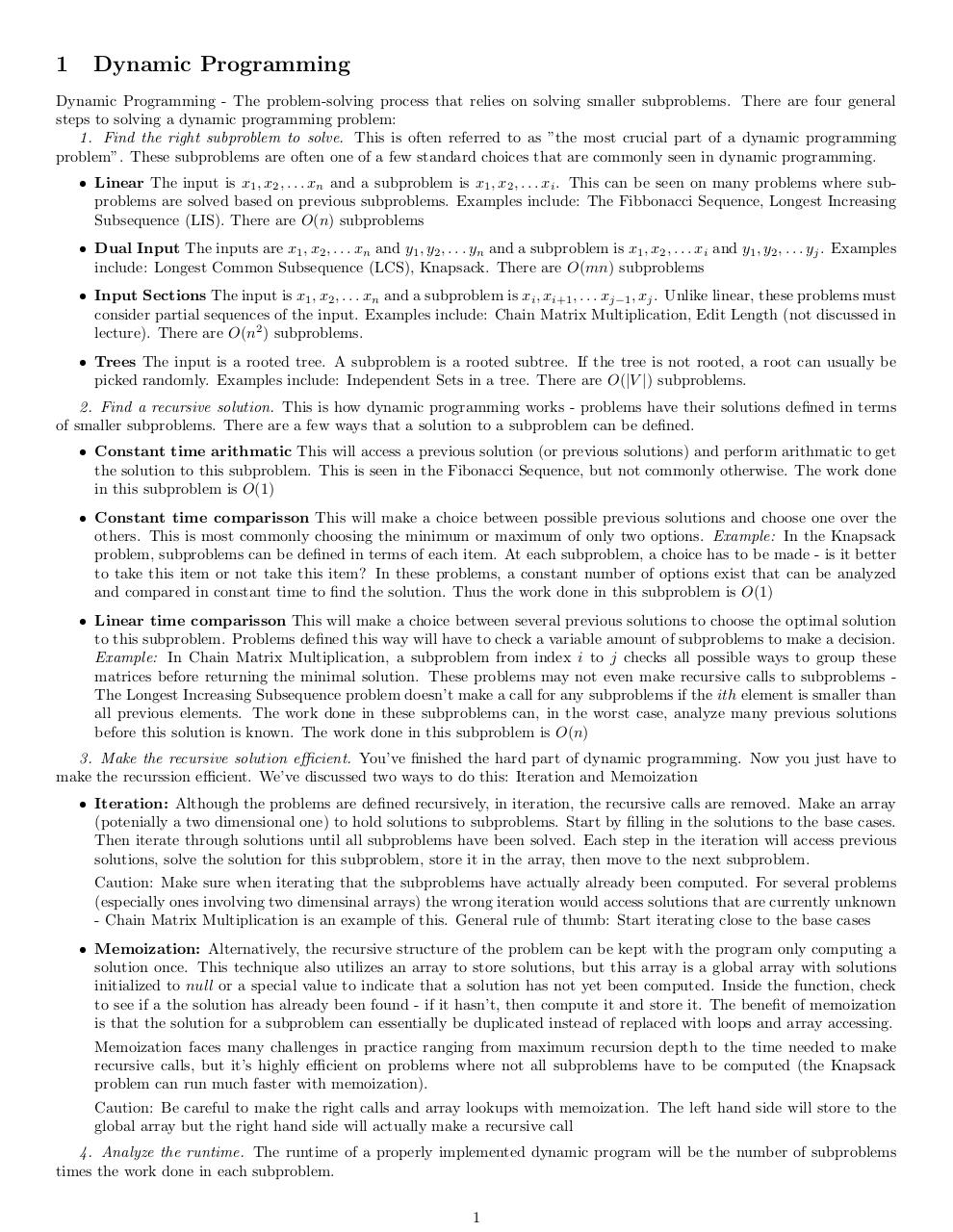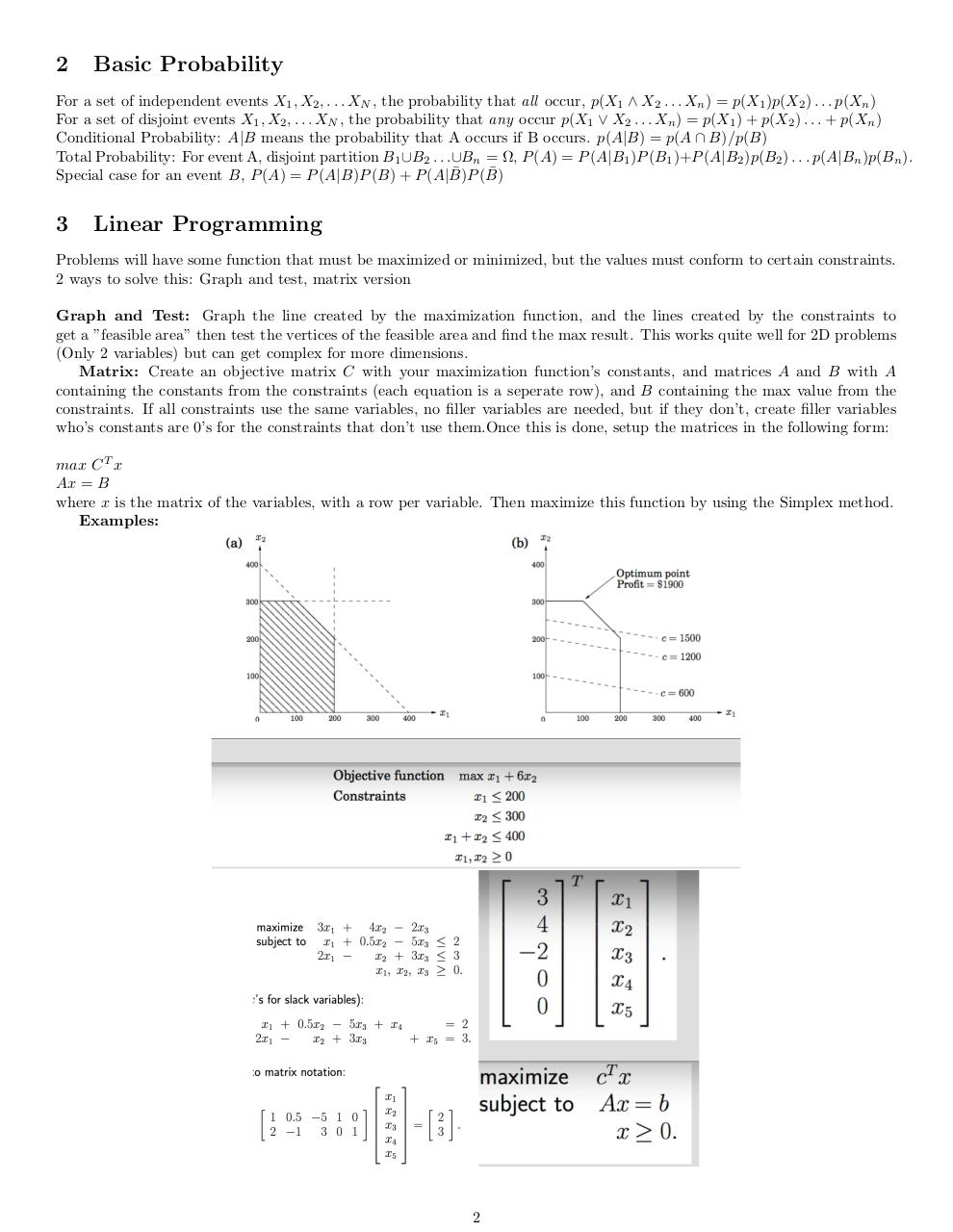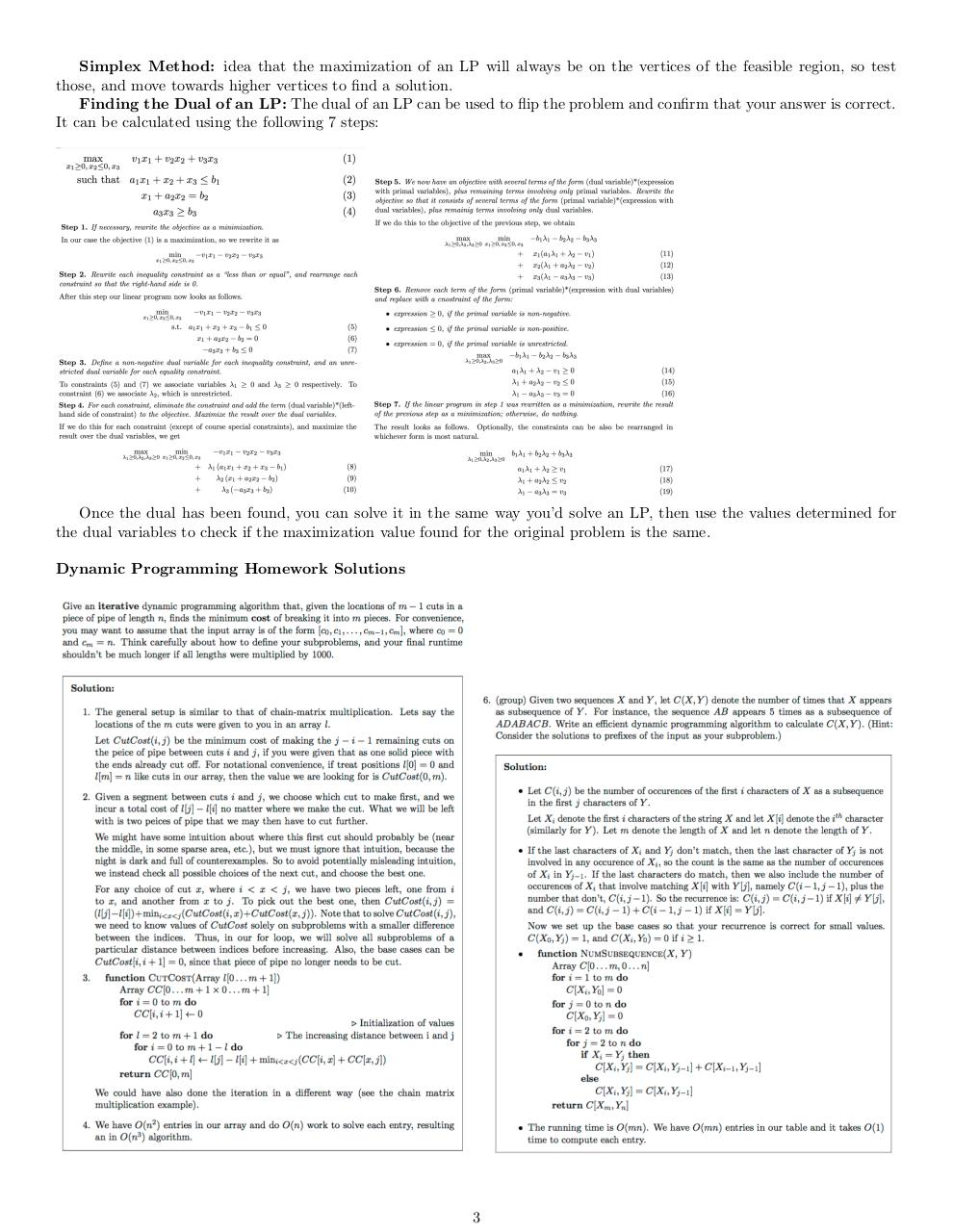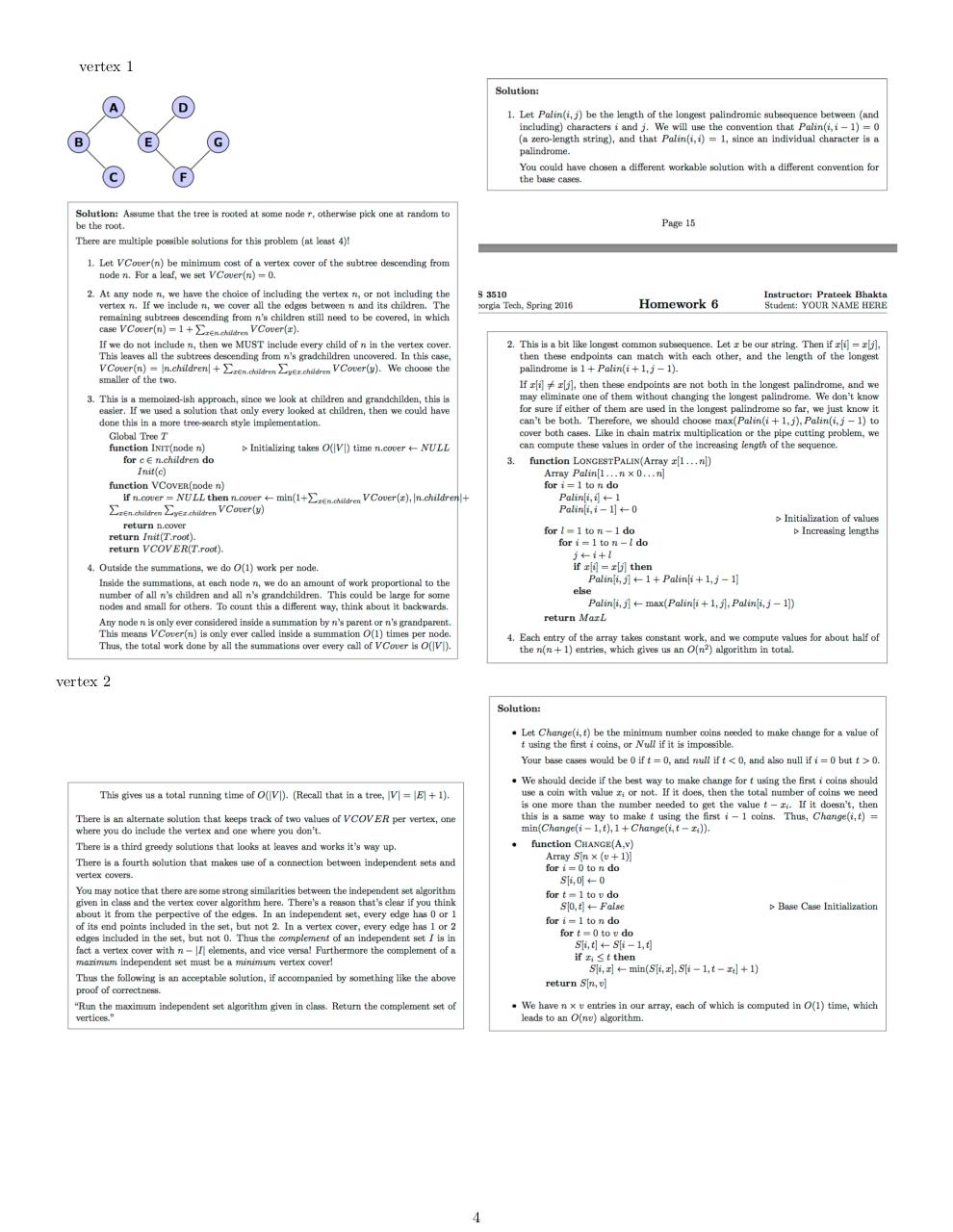# cs3510 test 3 cheat sheet .pdf

### File information

Original filename: cs3510-test-3-cheat-sheet.pdf

This PDF 1.5 document has been generated by TeX / pdfTeX-1.40.16, and has been sent on pdf-archive.com on 01/04/2016 at 12:30, from IP address 128.61.x.x. The current document download page has been viewed 664 times.
File size: 2.8 MB (4 pages).
Privacy: public file

cs3510-test-3-cheat-sheet.pdf (PDF, 2.8 MB)

### Document preview

1

Dynamic Programming

Dynamic Programming - The problem-solving process that relies on solving smaller subproblems. There are four general
steps to solving a dynamic programming problem:
1. Find the right subproblem to solve. This is often referred to as ”the most crucial part of a dynamic programming
problem”. These subproblems are often one of a few standard choices that are commonly seen in dynamic programming.
• Linear The input is x1 , x2 , . . . xn and a subproblem is x1 , x2 , . . . xi . This can be seen on many problems where subproblems are solved based on previous subproblems. Examples include: The Fibbonacci Sequence, Longest Increasing
Subsequence (LIS). There are O(n) subproblems
• Dual Input The inputs are x1 , x2 , . . . xn and y1 , y2 , . . . yn and a subproblem is x1 , x2 , . . . xi and y1 , y2 , . . . yj . Examples
include: Longest Common Subsequence (LCS), Knapsack. There are O(mn) subproblems
• Input Sections The input is x1 , x2 , . . . xn and a subproblem is xi , xi+1 , . . . xj−1 , xj . Unlike linear, these problems must
consider partial sequences of the input. Examples include: Chain Matrix Multiplication, Edit Length (not discussed in
lecture). There are O(n2 ) subproblems.
• Trees The input is a rooted tree. A subproblem is a rooted subtree. If the tree is not rooted, a root can usually be
picked randomly. Examples include: Independent Sets in a tree. There are O(|V |) subproblems.
2. Find a recursive solution. This is how dynamic programming works - problems have their solutions defined in terms
of smaller subproblems. There are a few ways that a solution to a subproblem can be defined.
• Constant time arithmatic This will access a previous solution (or previous solutions) and perform arithmatic to get
the solution to this subproblem. This is seen in the Fibonacci Sequence, but not commonly otherwise. The work done
in this subproblem is O(1)
• Constant time comparisson This will make a choice between possible previous solutions and choose one over the
others. This is most commonly choosing the minimum or maximum of only two options. Example: In the Knapsack
problem, subproblems can be defined in terms of each item. At each subproblem, a choice has to be made - is it better
to take this item or not take this item? In these problems, a constant number of options exist that can be analyzed
and compared in constant time to find the solution. Thus the work done in this subproblem is O(1)
• Linear time comparisson This will make a choice between several previous solutions to choose the optimal solution
to this subproblem. Problems defined this way will have to check a variable amount of subproblems to make a decision.
Example: In Chain Matrix Multiplication, a subproblem from index i to j checks all possible ways to group these
matrices before returning the minimal solution. These problems may not even make recursive calls to subproblems The Longest Increasing Subsequence problem doesn’t make a call for any subproblems if the ith element is smaller than
all previous elements. The work done in these subproblems can, in the worst case, analyze many previous solutions
before this solution is known. The work done in this subproblem is O(n)
3. Make the recursive solution efficient. You’ve finished the hard part of dynamic programming. Now you just have to
make the recurssion efficient. We’ve discussed two ways to do this: Iteration and Memoization
• Iteration: Although the problems are defined recursively, in iteration, the recursive calls are removed. Make an array
(potenially a two dimensional one) to hold solutions to subproblems. Start by filling in the solutions to the base cases.
Then iterate through solutions until all subproblems have been solved. Each step in the iteration will access previous
solutions, solve the solution for this subproblem, store it in the array, then move to the next subproblem.
Caution: Make sure when iterating that the subproblems have actually already been computed. For several problems
(especially ones involving two dimensinal arrays) the wrong iteration would access solutions that are currently unknown
- Chain Matrix Multiplication is an example of this. General rule of thumb: Start iterating close to the base cases
• Memoization: Alternatively, the recursive structure of the problem can be kept with the program only computing a
solution once. This technique also utilizes an array to store solutions, but this array is a global array with solutions
initialized to null or a special value to indicate that a solution has not yet been computed. Inside the function, check
to see if a the solution has already been found - if it hasn’t, then compute it and store it. The benefit of memoization
is that the solution for a subproblem can essentially be duplicated instead of replaced with loops and array accessing.
Memoization faces many challenges in practice ranging from maximum recursion depth to the time needed to make
recursive calls, but it’s highly efficient on problems where not all subproblems have to be computed (the Knapsack
problem can run much faster with memoization).
Caution: Be careful to make the right calls and array lookups with memoization. The left hand side will store to the
global array but the right hand side will actually make a recursive call
4. Analyze the runtime. The runtime of a properly implemented dynamic program will be the number of subproblems
times the work done in each subproblem.
1

2

Basic Probability

For a set of independent events X1 , X2 , . . . XN , the probability that all occur, p(X1 ∧ X2 . . . Xn ) = p(X1 )p(X2 ) . . . p(Xn )
For a set of disjoint events X1 , X2 , . . . XN , the probability that any occur p(X1 ∨ X2 . . . Xn ) = p(X1 ) + p(X2 ) . . . + p(Xn )
Conditional Probability: A|B means the probability that A occurs if B occurs. p(A|B) = p(A ∩ B)/p(B)
Total Probability: For event A, disjoint partition B1 ∪B2 . . .∪Bn = Ω, P (A) = P (A|B1 )P (B1 )+P (A|B2 )p(B2 ) . . . p(A|Bn )p(Bn ).
¯ (B)
¯
Special case for an event B, P (A) = P (A|B)P (B) + P (A|B)P

3

Linear Programming

Problems will have some function that must be maximized or minimized, but the values must conform to certain constraints.
2 ways to solve this: Graph and test, matrix version
Graph and Test: Graph the line created by the maximization function, and the lines created by the constraints to
get a ”feasible area” then test the vertices of the feasible area and find the max result. This works quite well for 2D problems
(Only 2 variables) but can get complex for more dimensions.
Matrix: Create an objective matrix C with your maximization function’s constants, and matrices A and B with A
containing the constants from the constraints (each equation is a seperate row), and B containing the max value from the
constraints. If all constraints use the same variables, no filler variables are needed, but if they don’t, create filler variables
who’s constants are 0’s for the constraints that don’t use them.Once this is done, setup the matrices in the following form:
max C T x
Ax = B
where x is the matrix of the variables, with a row per variable. Then maximize this function by using the Simplex method.
Examples:

2

Simplex Method: idea that the maximization of an LP will always be on the vertices of the feasible region, so test
those, and move towards higher vertices to find a solution.
Finding the Dual of an LP: The dual of an LP can be used to flip the problem and confirm that your answer is correct.
It can be calculated using the following 7 steps:

Once the dual has been found, you can solve it in the same way you’d solve an LP, then use the values determined for
the dual variables to check if the maximization value found for the original problem is the same.
Dynamic Programming Homework Solutions

3

vertex 1

vertex 2

4#### HTML Code

Copy the following HTML code to share your document on a Website or Blog

#### QR Code### Related keywords